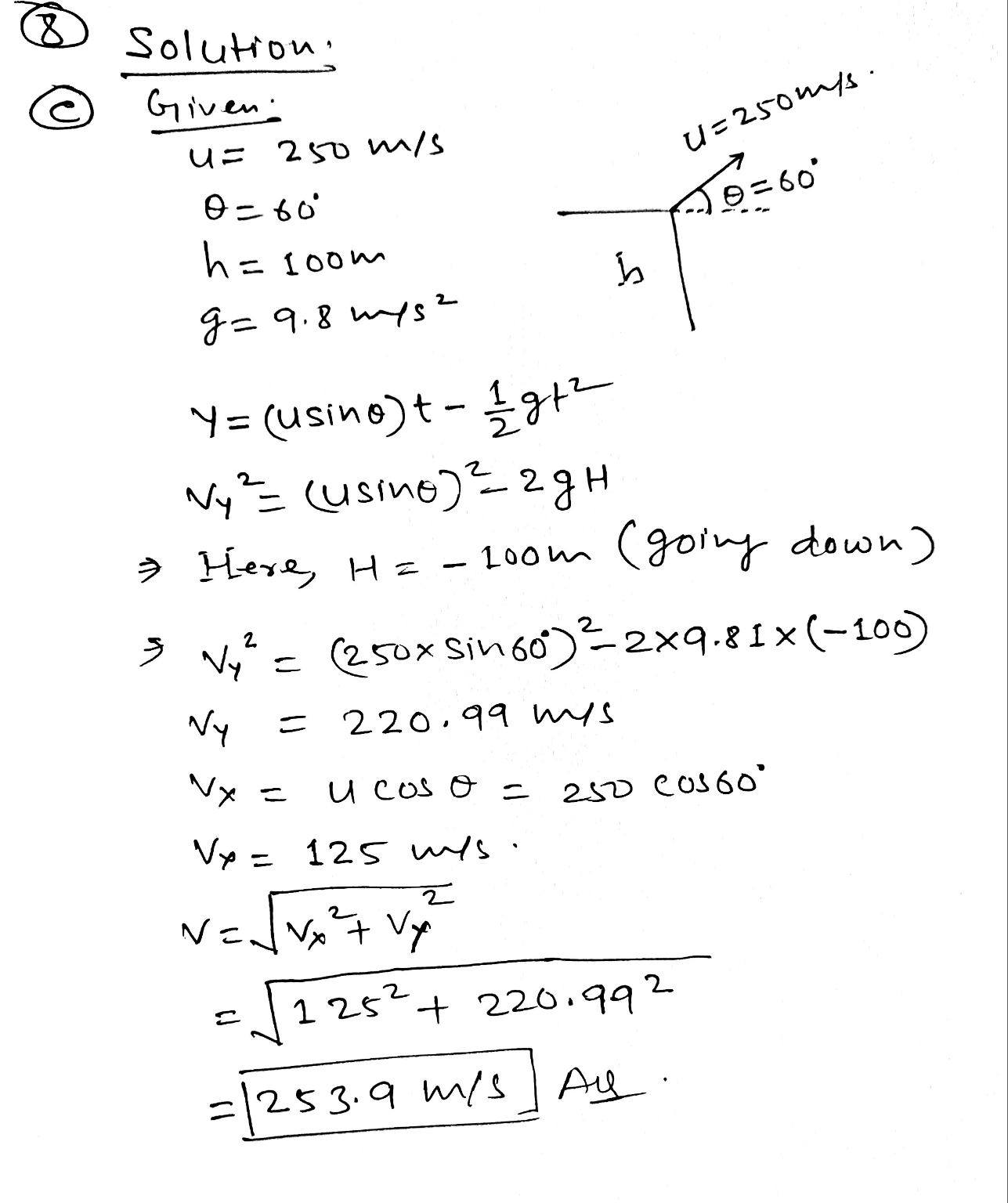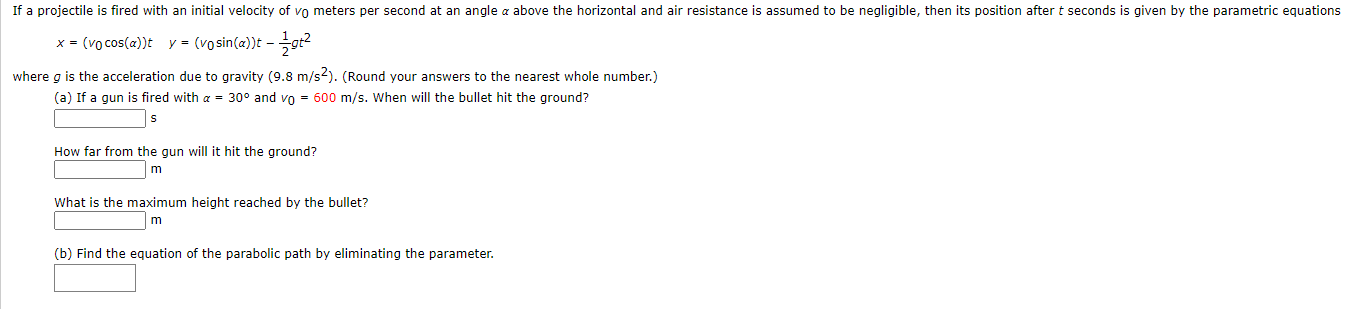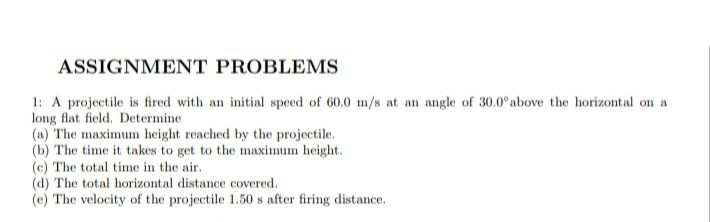Question

A projectile is fired with an initial speed of 250 m/s and angle of elevation 60°. The projectile is fired from a position 100 m above the ground. (Recall g ≈ 9.8 m/s2. Round your answers to the nearest whole number.)

(a) Find the range of the projectile.

(b) Find the maximum height reached.

(c) Find the speed at impact.#### Earn Coins

Coins can be redeemed for fabulous gifts.

Similar Homework Help Questions
• ### A projectile is fired with an initial speed of 250 m/s and angle of elevation 60°

A projectile is fired with an initial speed of 250 m/s and angle of elevation 60°. (Recall g ≈ 9.8 m/s2. Round your answers to the nearest whole number.) (a) Find the range of the projectile. (b) Find the maximum height reached. (c) Find the speed at impact.

• ### A projectile is fired with an initial speed of 20. m/s at an angle of 42.2°...

A projectile is fired with an initial speed of 20. m/s at an angle of 42.2° above the horizontal on a long flat firing range. Determine, a) the maximum height reached by the projectile, b) the total time in the air, c) the total horizontal distance covered (that is, the range), d) the speed of the projectile 1.50 s after firing. e) direction of the speed at 1.50 s.

• ### If a projectile is fired with an initial velocity of vo meters per second at an...If a projectile is fired with an initial velocity of vo meters per second at an angle a above the horizontal and air resistance is assumed to be negligible, then its position after t seconds is given by the parametric equations x = (vo cos(a)t y = (vo sin(a)t - +- 1012 where g is the acceleration due to gravity (9.8 m/s2). (Round your answers to the nearest whole number.) (a) If a gun is fired with a = 30°...

• ### A projectile is fired with an initial speed of 37.4 m/s at an angle of 45.0...

A projectile is fired with an initial speed of 37.4 m/s at an angle of 45.0 ° above the horizontal on a long flat firing range.Part ADetermine the maximum height reached by the projectile. Express your answer using three significant figures and include the appropriate units. Part B Determine the total time in the air Part C Determine the total horizontal distance covered (that is, the range).

• ### Projectile Motion: An object is fired into a projectile with an initial velocity of 250 m/s at an angle of 60° with resp...

Projectile Motion: An object is fired into a projectile with an initial velocity of 250 m/s at an angle of 60° with respect to the horizontal. a) What is the maximum height it reaches? b) What is the maximum horizontal distance it reaches? c) What is the time of flight of the particle? d) What is its velocity as it reaches the ground? e) Locate the object after 6 seconds.

• ### A projectile is fired with an initial speed of 36.2m/s at an angle of 41.1 degrees...

A projectile is fired with an initial speed of 36.2m/s at an angle of 41.1 degrees above the horizontal on a long flat firing range. determine maxiumum height reached by projectile total time in the air determine the range speed of the projectile 1.80s after firing

• ### A projectile is fired with an initial speed of 10 m/s. Find theangle of projection...

A projectile is fired with an initial speed of 10 m/s. Find the angle of projection such that the maximum height of the projectile is equal to 0.9 times its horizontal range.

• ### 1. If a projectile is fired with an initial velocity of vo meters per second at...

If a projectile is fired with an initial velocity of v0 meters per second at an angle α above the horizontal, and air resistance is assumed to be negligible, then its position after t seconds is given by the parametric equationsx=(v0 cos α) t   y=(v0 sin α) t-1/2 g t2where g is the acceleration due to gravity (9.8 m / s2)(a) If a projectile is fired with α=45° and v0=900 m / s, when will it hit the ground? How...

• ### ASSIGNMENT PROBLEMS 1: A projectile is fired with an initial speed of 60.0 m/s at an...ASSIGNMENT PROBLEMS 1: A projectile is fired with an initial speed of 60.0 m/s at an angle of 30.0°above the horizontal on a long flat field. Determine (a) The maximum height reached by the projectile. (b) The time it takes to get to the maximum height. (c) The total time in the air. (d) The total horizontal distance covered. (e) The velocity of the projectile 1.50 s after firing distance.